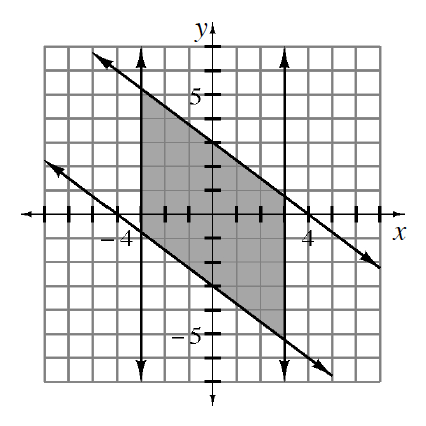### Home > INT3 > Chapter 5 > Lesson 5.1.2 > Problem5-31

5-31.

Write a system of inequalities that could be represented by the graph at right.

Use the slope and $y$-intercepts to write the equations of the diagonal lines. Vertical lines have equations that start with $x =$ .

Be sure to use the correct inequality symbols. One of your inequalities should be $x\ge−3$.